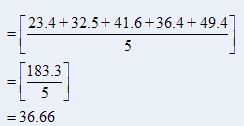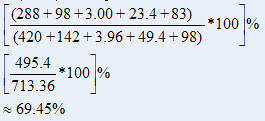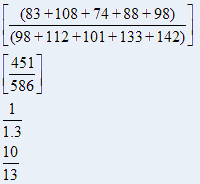Direction Study the following table and answer the questions based on it. Expenditures of a Company (in Pesetas) per Annum Over the given Years.1.

What is the average amount of interest per year which the company had to pay during this period?

 A. 32.43 B. 33.72 C. 34.18 D. 36.66
Explanation: Average amount of interest paid by the Company during the given period2.

The total amount of bonus paid by the company during the given period is approximately what percent of the total amount of salary paid during this period?

 A. 0.1% B. 0.5% C. 1.0% D. 1.25%

Explanation: Required percentage3.

Total expenditure on all these items in 1998 was approximately what percent of the total expenditure in 2002?

 A. 62% B. 66% C. 69% D. 71%

Explanation: Required percentage4.

The total expenditure of the company over these items during the year 2000 is?

 A. 544.44 B. 501.11 C. 446.46 D. 478.87

Explanation: Total expenditure of the Company during 2000 = (324 + 101 + 3.84 + 41.6 + 74) pesetas = 544.44 pesetas

5.

The ratio between the total expenditure on Taxes for all the years and the total expenditure on Fuel and Transport for all the years respectively is approximately?

 A. 4:7 B. 10:13 C. 15:18 D. 5:8